# 21.E: Organic Chemistry (Exercises)

•• OpenStax
• OpenStax
$$\newcommand{\vecs}{\overset { \rightharpoonup} {\mathbf{#1}} }$$ $$\newcommand{\vecd}{\overset{-\!-\!\rightharpoonup}{\vphantom{a}\smash {#1}}}$$$$\newcommand{\id}{\mathrm{id}}$$ $$\newcommand{\Span}{\mathrm{span}}$$ $$\newcommand{\kernel}{\mathrm{null}\,}$$ $$\newcommand{\range}{\mathrm{range}\,}$$ $$\newcommand{\RealPart}{\mathrm{Re}}$$ $$\newcommand{\ImaginaryPart}{\mathrm{Im}}$$ $$\newcommand{\Argument}{\mathrm{Arg}}$$ $$\newcommand{\norm}{\| #1 \|}$$ $$\newcommand{\inner}{\langle #1, #2 \rangle}$$ $$\newcommand{\Span}{\mathrm{span}}$$ $$\newcommand{\id}{\mathrm{id}}$$ $$\newcommand{\Span}{\mathrm{span}}$$ $$\newcommand{\kernel}{\mathrm{null}\,}$$ $$\newcommand{\range}{\mathrm{range}\,}$$ $$\newcommand{\RealPart}{\mathrm{Re}}$$ $$\newcommand{\ImaginaryPart}{\mathrm{Im}}$$ $$\newcommand{\Argument}{\mathrm{Arg}}$$ $$\newcommand{\norm}{\| #1 \|}$$ $$\newcommand{\inner}{\langle #1, #2 \rangle}$$ $$\newcommand{\Span}{\mathrm{span}}$$$$\newcommand{\AA}{\unicode[.8,0]{x212B}}$$

## 20.1: Hydrocarbons

### Q20.1.1

Write the chemical formula and Lewis structure of the following, each of which contains five carbon atoms:

1. an alkane
2. an alkene
3. an alkyne

### S20.1.1

There are several sets of answers; one is:

(a) C5H12(b) C5H10(c) C5H8### Q20.1.2

What is the difference between the hybridization of carbon atoms’ valence orbitals in saturated and unsaturated hydrocarbons?

### Q20.1.3

On a microscopic level, how does the reaction of bromine with a saturated hydrocarbon differ from its reaction with an unsaturated hydrocarbon? How are they similar?

### Q20.1.4

Both reactions result in bromine being incorporated into the structure of the product. The difference is the way in which that incorporation takes place. In the saturated hydrocarbon, an existing C–H bond is broken, and a bond between the C and the Br can then be formed. In the unsaturated hydrocarbon, the only bond broken in the hydrocarbon is the π bond whose electrons can be used to form a bond to one of the bromine atoms in Br2 (the electrons from the Br–Br bond form the other C–Br bond on the other carbon that was part of the π bond in the starting unsaturated hydrocarbon).

### Q20.1.5

On a microscopic level, how does the reaction of bromine with an alkene differ from its reaction with an alkyne? How are they similar?

### Q20.1.6

Explain why unbranched alkenes can form geometric isomers while unbranched alkanes cannot. Does this explanation involve the macroscopic domain or the microscopic domain?

### S20.1.6

Unbranched alkanes have free rotation about the C–C bonds, yielding all orientations of the substituents about these bonds equivalent, interchangeable by rotation. In the unbranched alkenes, the inability to rotate about the $$\mathrm{C=C}$$ bond results in fixed (unchanging) substituent orientations, thus permitting different isomers. Since these concepts pertain to phenomena at the molecular level, this explanation involves the microscopic domain.

Explain why these two molecules are not isomers:### Q20.1.7

Explain why these two molecules are not isomers:### S20.1.6

They are the same compound because each is a saturated hydrocarbon containing an unbranched chain of six carbon atoms.

### Q20.1.7

How does the carbon-atom hybridization change when polyethylene is prepared from ethylene?

### Q20.1.8

Write the Lewis structure and molecular formula for each of the following hydrocarbons:

1. hexane
2. 3-methylpentane
3. cis-3-hexene
4. 4-methyl-1-pentene
5. 3-hexyne
6. 4-methyl-2-pentyne

### Q20.1.9

(a) C6H14(b) C6H14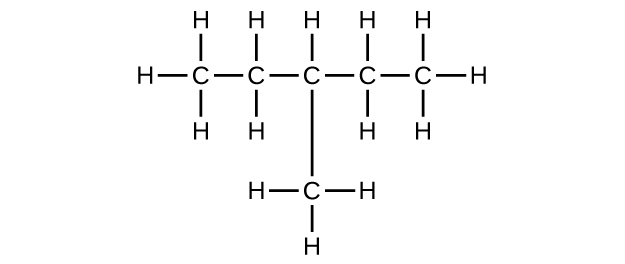(c) C6H12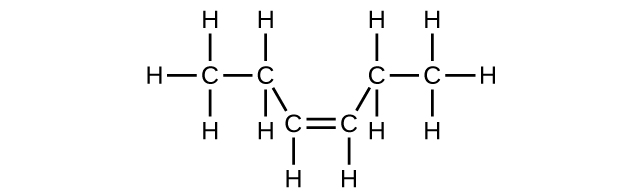(d) C6H12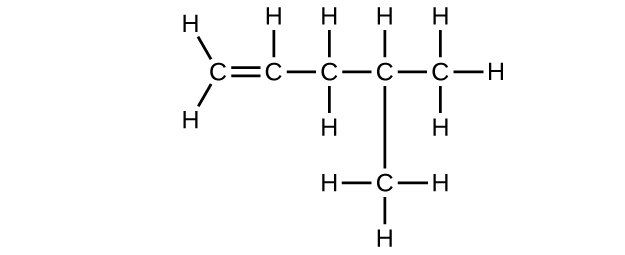(e) C6H10(f) C6H10### Q20.1.10

Write the chemical formula, condensed formula, and Lewis structure for each of the following hydrocarbons:

1. heptane
2. 3-methylhexane
3. trans-3-heptene
4. 4-methyl-1-hexene
5. 2-heptyne
6. 3,4-dimethyl-1-pentyne

### Q20.1.11

Give the complete IUPAC name for each of the following compounds:

1. CH3CH2CBr2CH3
2. (CH3)3CCl
3.4. $$\mathrm{CH_3CH_2C≡CH\, CH_3CH_2C≡CH}\ 5.6.7. \(\mathrm{(CH_3)_2CHCH_2CH=CH_2}$$

### S20.1.11

(a) 2,2-dibromobutane; (b) 2-chloro-2-methylpropane; (c) 2-methylbutane; (d) 1-butyne; (e) 4-fluoro-4-methyl-1-octyne; (f) trans-1-chloropropene; (g) 5-methyl-1-pentene

### Q20.1.12

Give the complete IUPAC name for each of the following compounds:

1. (CH3)2CHF
2. CH3CHClCHClCH3
3.4. $$\mathrm{CH_3CH_2CH=CHCH_3}$$
5.6. $$\mathrm{(CH_3)_3CCH_2C≡CH}$$

### Q20.1.13

Butane is used as a fuel in disposable lighters. Write the Lewis structure for each isomer of butane.

### S20.1.13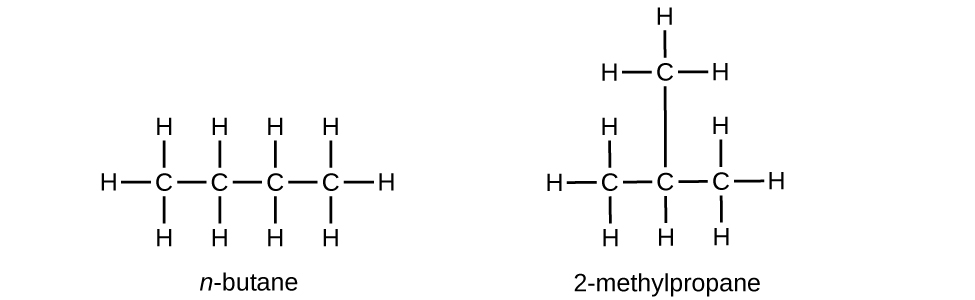### Q20.1.14

Write Lewis structures and name the five structural isomers of hexane.

### Q20.1.15

Write Lewis structures for the cis–trans isomers of $$\mathrm{CH_3CH=CHCl}$$.

### S20.1.15### Q20.1.16

Write structures for the three isomers of the aromatic hydrocarbon xylene, C6H4(CH3)2.

### Q20.1.17

Isooctane is the common name of the isomer of C8H18 used as the standard of 100 for the gasoline octane rating:1. What is the IUPAC name for the compound?
2. Name the other isomers that contain a five-carbon chain with three methyl substituents.

### Q20.1.18

(a) 2,2,4-trimethylpentane; (b) 2,2,3-trimethylpentane, 2,3,4-trimethylpentane, and 2,3,3-trimethylpentane: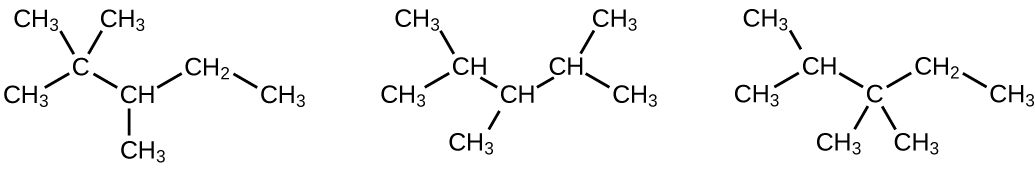### Q20.1.19

Write Lewis structures and IUPAC names for the alkyne isomers of C4H6.

### Q20.1.20

Write Lewis structures and IUPAC names for all isomers of C4H9Cl.

### S20.1.20### Q20.1.21

Name and write the structures of all isomers of the propyl and butyl alkyl groups.

### Q20.1.22

Write the structures for all the isomers of the –C5H11 alkyl group.

### Q20.1.23

In the following, the carbon backbone and the appropriate number of hydrogen atoms are shown in condensed form:### Q20.1.24

Write Lewis structures and describe the molecular geometry at each carbon atom in the following compounds:

1. cis-3-hexene
2. cis-1-chloro-2-bromoethene
3. 2-pentyne
4. trans-6-ethyl-7-methyl-2-octene

### Q20.1.25

Benzene is one of the compounds used as an octane enhancer in unleaded gasoline. It is manufactured by the catalytic conversion of acetylene to benzene:

$\ce{3C2H2 ⟶ C6H6}$

Draw Lewis structures for these compounds, with resonance structures as appropriate, and determine the hybridization of the carbon atoms in each.

### S20.1.25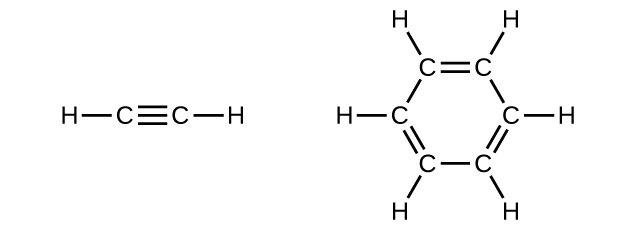### Q20.1.26

In acetylene, the bonding uses sp hybrids on carbon atoms and s orbitals on hydrogen atoms. In benzene, the carbon atoms are sp2 hybridized.

### Q20.1.27

Teflon is prepared by the polymerization of tetrafluoroethylene. Write the equation that describes the polymerization using Lewis symbols.

### Q20.1.28

Write two complete, balanced equations for each of the following reactions, one using condensed formulas and one using Lewis structures.

1. 1 mol of 1-butyne reacts with 2 mol of iodine.
2. Pentane is burned in air.

### S20.1.28

(a) $$\mathrm{CH=CCH_2CH_3 + 2I_2⟶ CHI_2CI_2CH_2CH_3}$$(b) $$\ce{CH3CH2CH2CH2CH3 + 8O2 ⟶ 5CO2 + 6H2O}$$### Q20.1.29

Write two complete, balanced equations for each of the following reactions, one using condensed formulas and one using Lewis structures.

1. 2-butene reacts with chlorine.
2. benzene burns in air.

### Q20.1.30

What mass of 2-bromopropane could be prepared from 25.5 g of propene? Assume a 100% yield of product.

65.2 g

### Q20.1.31

Acetylene is a very weak acid; however, it will react with moist silver(I) oxide and form water and a compound composed of silver and carbon. Addition of a solution of HCl to a 0.2352-g sample of the compound of silver and carbon produced acetylene and 0.2822 g of AgCl.

1. What is the empirical formula of the compound of silver and carbon?
2. The production of acetylene on addition of HCl to the compound of silver and carbon suggests that the carbon is present as the acetylide ion, $$\ce{C2^2-}$$. Write the formula of the compound showing the acetylide ion.

### Q20.1.32

Ethylene can be produced by the pyrolysis of ethane:

$$\ce{C2H6⟶C2H4 + H2}$$

How many kilograms of ethylene is produced by the pyrolysis of 1.000 × 103 kg of ethane, assuming a 100.0% yield?

9.328 × 102 kg

## 20.2: Alcohols and Ethers

Why do the compounds hexane, hexanol, and hexene have such similar names?

Write condensed formulas and provide IUPAC names for the following compounds:

1. (a) ethyl alcohol (in beverages)
2. (b) methyl alcohol (used as a solvent, for example, in shellac)
3. (c) ethylene glycol (antifreeze)
4. (d) isopropyl alcohol (used in rubbing alcohol)
5. (e) glycerine

(a) ethyl alcohol, ethanol: CH3CH2OH; (b) methyl alcohol, methanol: CH3OH; (c) ethylene glycol, ethanediol: HOCH2CH2OH; (d) isopropyl alcohol, 2-propanol: CH3CH(OH)CH3; (e) glycerine, l,2,3-trihydroxypropane: HOCH2CH(OH)CH2OH

Give the complete IUPAC name for each of the following compounds:

(a)(b)(c)Give the complete IUPAC name and the common name for each of the following compounds:

(a)(b)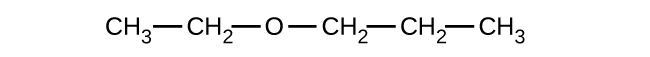(c)(a) 1-ethoxybutane, butyl ethyl ether; (b) 1-ethoxypropane, ethyl propyl ether; (c) 1-methoxypropane, methyl propyl ether

Write the condensed structures of both isomers with the formula C2H6O. Label the functional group of each isomer.

Write the condensed structures of all isomers with the formula C2H6O2. Label the functional group (or groups) of each isomer.

HOCH2CH2OH, two alcohol groups; CH3OCH2OH, ether and alcohol groups

Draw the condensed formulas for each of the following compounds:

1. (a) dipropyl ether
2. (b) 2,2-dimethyl-3-hexanol
3. (c) 2-ethoxybutane

MTBE, Methyl tert-butyl ether, CH3OC(CH3)3, is used as an oxygen source in oxygenated gasolines. MTBE is manufactured by reacting 2-methylpropene with methanol.

1. (a) Using Lewis structures, write the chemical equation representing the reaction.
2. (b) What volume of methanol, density 0.7915 g/mL, is required to produce exactly 1000 kg of MTBE, assuming a 100% yield?

(a)(b) 4.593 × 102 L

Write two complete balanced equations for each of the following reactions, one using condensed formulas and one using Lewis structures.

(a) propanol is converted to dipropyl ether

(b) propene is treated with water in dilute acid.

Write two complete balanced equations for each of the following reactions, one using condensed formulas and one using Lewis structures.

1. (a) 2-butene is treated with water in dilute acid
2. (b) ethanol is dehydrated to yield ethene

(a) $$\mathrm{CH_3CH=CHCH_3+H_2O⟶CH_3CH_2CH(OH)CH_3}$$

;(b) $$\mathrm{CH_3CH_2OH⟶CH_2=CH_2+H_2O}$$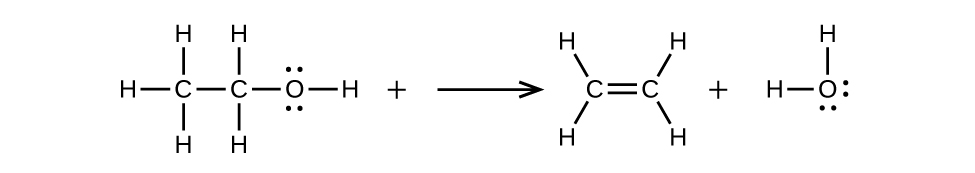## 20.3: Aldehydes, Ketones, Carboxylic Acids, and Esters

### Q20.3.1

Order the following molecules from least to most oxidized, based on the marked carbon atom: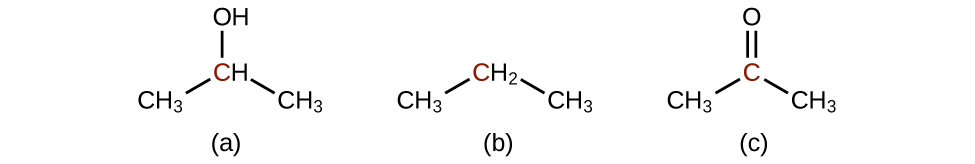### Q20.3.2

Predict the products of oxidizing the molecules shown in this problem. In each case, identify the product that will result from the minimal increase in oxidation state for the highlighted carbon atom:

(a)(b)(c)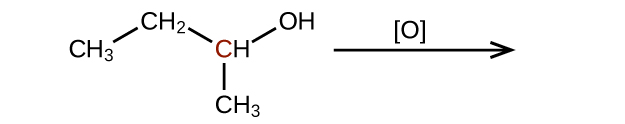(a)(b)(c)### Q20.3.3

Predict the products of reducing the following molecules. In each case, identify the product that will result from the minimal decrease in oxidation state for the highlighted carbon atom:

(a)(b)(c)### Q20.3.4

Explain why it is not possible to prepare a ketone that contains only two carbon atoms.

### S20.3.4

A ketone contains a group bonded to two additional carbon atoms; thus, a minimum of three carbon atoms are needed.

### Q20.3.5

How does hybridization of the substituted carbon atom change when an alcohol is converted into an aldehyde? An aldehyde to a carboxylic acid?

### Q20.3.6

Fatty acids are carboxylic acids that have long hydrocarbon chains attached to a carboxylate group. How does a saturated fatty acid differ from an unsaturated fatty acid? How are they similar?

### S20.3.6

Since they are both carboxylic acids, they each contain the –COOH functional group and its characteristics. The difference is the hydrocarbon chain in a saturated fatty acid contains no double or triple bonds, whereas the hydrocarbon chain in an unsaturated fatty acid contains one or more multiple bonds.

### S20.3.7

Write a condensed structural formula, such as CH3CH3, and describe the molecular geometry at each carbon atom.

1. propene
2. 1-butanol
3. ethyl propyl ether
4. cis-4-bromo-2-heptene
5. 2,2,3-trimethylhexane
6. formaldehyde

### Q20.3.8

Write a condensed structural formula, such as CH3CH3, and describe the molecular geometry at each carbon atom.

1. 2-propanol
2. acetone
3. dimethyl ether
4. acetic acid
5. 3-methyl-1-hexene

### S20.3.8

(a) CH3CH(OH)CH3: all carbons are tetrahedral; (b) $$\ce{CH3C(==O)CH3}$$: the end carbons are tetrahedral and the central carbon is trigonal planar; (c) CH3OCH3: all are tetrahedral; (d) CH3COOH: the methyl carbon is tetrahedral and the acid carbon is trigonal planar; (e) CH3CH2CH2CH(CH3)CHCH2: all are tetrahedral except the right-most two carbons, which are trigonal planar

### Q20.3.9

The foul odor of rancid butter is caused by butyric acid, CH3CH2CH2CO2H.

1. Draw the Lewis structure and determine the oxidation number and hybridization for each carbon atom in the molecule.
2. The esters formed from butyric acid are pleasant-smelling compounds found in fruits and used in perfumes. Draw the Lewis structure for the ester formed from the reaction of butyric acid with 2-propanol.

### Q20.3.10

Write the two-resonance structures for the acetate ion.### Q20.3.11

Write two complete, balanced equations for each of the following reactions, one using condensed formulas and one using Lewis structures:

1. ethanol reacts with propionic acid
2. benzoic acid, C6H5CO2H, is added to a solution of sodium hydroxide

### Q20.3.12

Write two complete balanced equations for each of the following reactions, one using condensed formulas and one using Lewis structures.

1. 1-butanol reacts with acetic acid
2. propionic acid is poured onto solid calcium carbonate

### S20.3.12

(a) $$\ce{CH3CH2CH2CH2OH + CH3C(O)OH⟶CH3C(O)OCH2CH2CH2CH3 + H2O}$$:(b) $$\ce{2CH3CH2COOH + CaCO3⟶(CH3CH2COO)2Ca + CO2 + H2O}$$:### Q20.3.13

Yields in organic reactions are sometimes low. What is the percent yield of a process that produces 13.0 g of ethyl acetate from 10.0 g of CH3CO2H?

### Q20.3.14

Alcohols A, B, and C all have the composition C4H10O. Molecules of alcohol A contain a branched carbon chain and can be oxidized to an aldehyde; molecules of alcohol B contain a linear carbon chain and can be oxidized to a ketone; and molecules of alcohol C can be oxidized to neither an aldehyde nor a ketone. Write the Lewis structures of these molecules.

### S20.3.15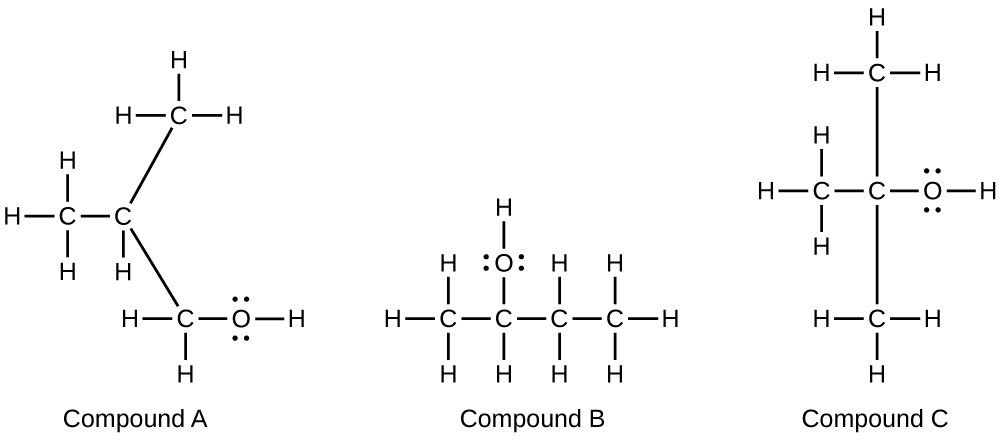## 20.4: Amines and Amides

### Q20.4.1

Write the Lewis structures of both isomers with the formula C2H7N.

### Q20.4.2

What is the molecular structure about the nitrogen atom in trimethyl amine and in the trimethyl ammonium ion, (CH3)3NH+? What is the hybridization of the nitrogen atom in trimethyl amine and in the trimethyl ammonium ion?

### S20.4.2

Trimethyl amine: trigonal pyramidal, sp3; trimethyl ammonium ion: tetrahedral, sp3

### Q20.4.3

Write the two resonance structures for the pyridinium ion, C5H5NH+.

### Q20.4.4

Draw Lewis structures for pyridine and its conjugate acid, the pyridinium ion, C5H5NH+. What are the geometries and hybridizations about the nitrogen atoms in pyridine and in the pyridinium ion?### Q20.4.5

Write the Lewis structures of all isomers with the formula C3H7ON that contain an amide linkage.

### Q20.4.6

Write two complete balanced equations for the following reaction, one using condensed formulas and one using Lewis structures.

• Methyl amine is added to a solution of HCl.

### S20.4.6

$\ce{CH3NH2 + H3O+ ⟶CH3NH3+ + H2O}$### Q20.4.7

Write two complete, balanced equations for each of the following reactions, one using condensed formulas and one using Lewis structures.

Ethylammonium chloride is added to a solution of sodium hydroxide.

### Q20.4.8

Identify any carbon atoms that change hybridization and the change in hybridization during the reactions in [link].

### S20.4.8

CH3CH = CHCH3(sp2) + Cl $$⟶$$ CH3CH(Cl)H(Cl)CH3(sp3);

2C6H6(sp2) + 15O2 $$⟶$$ 12CO2(sp) + 6H2O

### Q20.4.9

Identify any carbon atoms that change hybridization and the change in hybridization during the reactions in [link].

### Q20.4.10

Identify any carbon atoms that change hybridization and the change in hybridization during the reactions in [link].

### S20.4.10

the carbon in CO32−, initially at sp2, changes hybridization to sp in CO2

This page titled 21.E: Organic Chemistry (Exercises) is shared under a CC BY license and was authored, remixed, and/or curated by OpenStax.